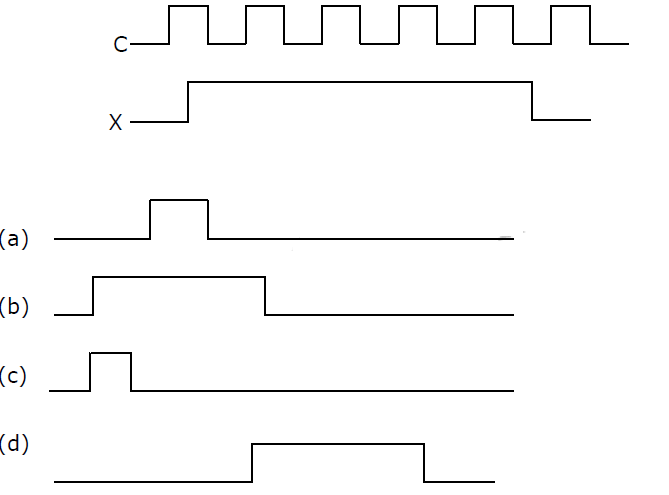# GATE | GATE-CS-2001 | Question 33

• Difficulty Level : Medium
• Last Updated : 12 Dec, 2018

Consider the following circuit with initial state Q0 = Q1 = 0. The D Flip-flops are positive edged triggered and have set up times 20 nanosecond and hold times 0.Attention reader! Don’t stop learning now.  Practice GATE exam well before the actual exam with the subject-wise and overall quizzes available in GATE Test Series Course.

Learn all GATE CS concepts with Free Live Classes on our youtube channel.

Consider the following timing diagrams of X and C; the clock period of C <= 40 nanosecond. Which one is the correct plot of Y?(A) a
(B) b
(C) c
(D) d

Explanation: Set up time and hold times are given just to ensure that edge triggering works properly.

1. Since clock is positive edge triggered, so first positive edge trigger: Since X is 0, so output will be 0. Also, Q0 and Q0′ are 0 and 1 respectively.
2. Now, in second step, Q0′ would be 1 because of setup time of flip-flop is 20 ns and clock-period is ≥ 40 ns.
Therefore, second positive edge trigger: Because of X is 1 and Q0′ is 1, so output is 1.
3. Now, Q0′ will became 0, but output Y won’t change as the flip-flop is positive edge triggered.
Third positive edge trigger: Because of X is 1 and Q0′ is 0, so output is 0.
4. Now, output never changes back to 1 as Q0′ is always 0 and when Q0′ finally becomes 1, X is 0.

So, option (A) is correct.

Please comment below if you find anything wrong in the above post.

Quiz of this Question

My Personal Notes arrow_drop_up